# This Python 3 environment comes with many helpful analytics libraries installed
# It is defined by the kaggle/python docker image: https://github.com/kaggle/docker-python

import numpy as np # linear algebra
import pandas as pd # data processing, CSV file I/O (e.g. pd.read_csv)

# Input data files are available in the "../input/" directory.
# For example, running this (by clicking run or pressing Shift+Enter) will list all files under the input directory

import os
for dirname, _, filenames in os.walk('/kaggle/input'):
for filename in filenames:
print(os.path.join(dirname, filename))

# Any results you write to the current directory are saved as output.

/kaggle/input/factors-affecting-campus-placement/Placement_Data_Full_Class.csv

#Lets import important libraries
import seaborn as sns
import matplotlib.pyplot as plt
from sklearn.preprocessing import LabelEncoder
%matplotlib inline

#Fetching file into a dataframe

#Lets understand data

sl_no gender ssc_p ssc_b hsc_p hsc_b hsc_s degree_p degree_t workex etest_p specialisation mba_p status salary
0 1 M 67.00 Others 91.00 Others Commerce 58.00 Sci&Tech No 55.0 Mkt&HR 58.80 Placed 270000.0
1 2 M 79.33 Central 78.33 Others Science 77.48 Sci&Tech Yes 86.5 Mkt&Fin 66.28 Placed 200000.0
2 3 M 65.00 Central 68.00 Central Arts 64.00 Comm&Mgmt No 75.0 Mkt&Fin 57.80 Placed 250000.0
3 4 M 56.00 Central 52.00 Central Science 52.00 Sci&Tech No 66.0 Mkt&HR 59.43 Not Placed NaN
4 5 M 85.80 Central 73.60 Central Commerce 73.30 Comm&Mgmt No 96.8 Mkt&Fin 55.50 Placed 425000.0
df.info()

<class 'pandas.core.frame.DataFrame'>
RangeIndex: 215 entries, 0 to 214
Data columns (total 15 columns):
sl_no             215 non-null int64
gender            215 non-null object
ssc_p             215 non-null float64
ssc_b             215 non-null object
hsc_p             215 non-null float64
hsc_b             215 non-null object
hsc_s             215 non-null object
degree_p          215 non-null float64
degree_t          215 non-null object
workex            215 non-null object
etest_p           215 non-null float64
specialisation    215 non-null object
mba_p             215 non-null float64
status            215 non-null object
salary            148 non-null float64
dtypes: float64(6), int64(1), object(8)
memory usage: 25.3+ KB

df.isnull().sum()

sl_no              0
gender             0
ssc_p              0
ssc_b              0
hsc_p              0
hsc_b              0
hsc_s              0
degree_p           0
degree_t           0
workex             0
etest_p            0
specialisation     0
mba_p              0
status             0
salary            67
dtype: int64

Salary column has 67 null values .Need to fix this.Lets replace null values by 0.

df['salary'].fillna(0,inplace = True)

df.isnull().sum()

sl_no             0
gender            0
ssc_p             0
ssc_b             0
hsc_p             0
hsc_b             0
hsc_s             0
degree_p          0
degree_t          0
workex            0
etest_p           0
specialisation    0
mba_p             0
status            0
salary            0
dtype: int64

Now our data is preprocessed and ready for exploration.

#Count of males and females in data
df_gender_analysis =  df[['gender','status']].groupby(['gender'], as_index = False).count()
df_gender_analysis1 = df.loc[df['status'] == 'Placed'].groupby(['gender'],as_index = False).count()
df_gender_analysis['Placed'] = df_gender_analysis1['status']
df_gender_analysis['Placed_Percent'] = df_gender_analysis['Placed']/df_gender_analysis['status']*100
df_gender_analysis.rename(columns = {'gender':'Gender' , 'status':'Total_Students'})

Gender Total_Students Placed Placed_Percent
0 F 76 48 63.157895
1 M 139 100 71.942446

Percentage of Male students is more than that of Females . Male students got placed more as compared to females.

#Salary analysis by gender
df_gender_analysis2 = df[['gender','salary']].groupby(['gender'],as_index = False).mean()
df_gender_analysis2

gender salary
0 F 168815.789474
1 M 215043.165468

Here we can see that male students have more mean salary as compared to females

#Lets see salary distribution
plt.figure(figsize=(12,9))
df_male = df.loc[df['gender'] == 'M']
ax = sns.distplot(df_male['salary'].loc[df['salary']!=0])
ax.ticklabel_format(style = 'plain')
plt.show()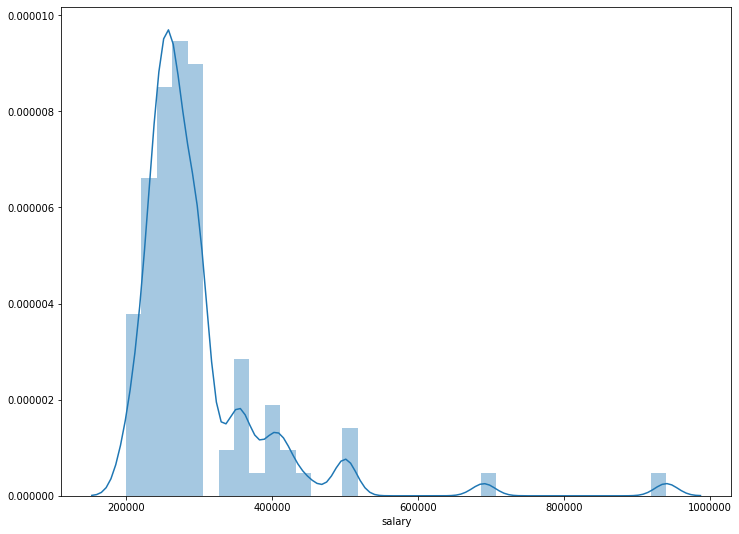Mostly people got salaries in between 200k to 400k.

#Lets see salary distribution by gender.
fig, ax =plt.subplots(1,2 , figsize = (14,7))
df_male = df.loc[df['gender'] == 'M']
df_female = df.loc[df['gender'] == 'F']
sns.distplot(df_male['salary'].loc[df['salary']!=0] , ax = ax)
sns.distplot(df_female['salary'].loc[df['salary']!=0] , ax = ax)
ax.ticklabel_format(style = 'plain')
ax.ticklabel_format(style = 'plain')
ax.set_title("Male")
ax.set_title("Female")
plt.show()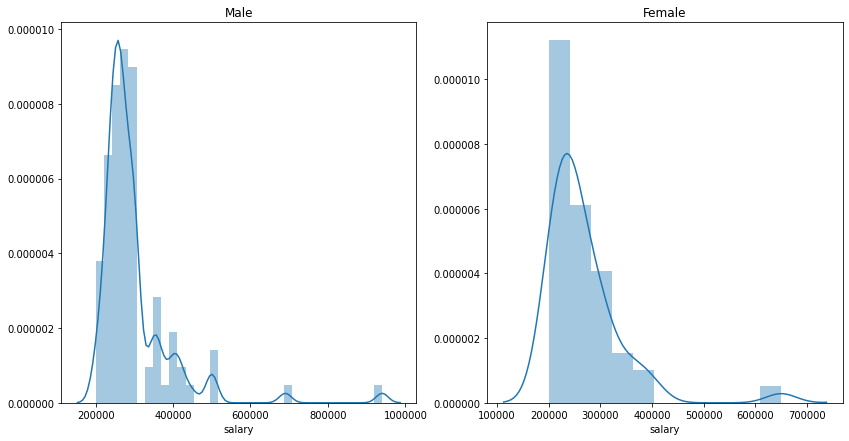Deviation is less for males than compared to females which means most people get salary close to mean salary.

sns.countplot(x = 'workex' , data =df , hue = 'status')

<matplotlib.axes._subplots.AxesSubplot at 0x7eff527ab358>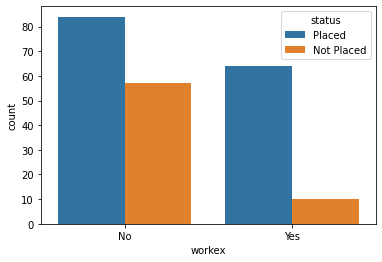From the graph there is huge difference between placed count and unplaced for people having work expereince.That means with work experience you are more likely to get placed as compared to wth no work experience.Work Experience is an imortant factor for your placement.It increases your chances of getting a placement.Now lets see the impact of work experience on salary.

#Lets see mean salaries for workex and no workex
df[['workex','salary']].groupby(['workex']).mean()

salary
workex
No 165333.333333
Yes 262283.783784

Work ex mean salary is higher as expected

sns.boxplot(x = 'workex' , y = 'salary' , data=df.loc[df['status'] == 'Placed'])

<matplotlib.axes._subplots.AxesSubplot at 0x7eff52817ba8>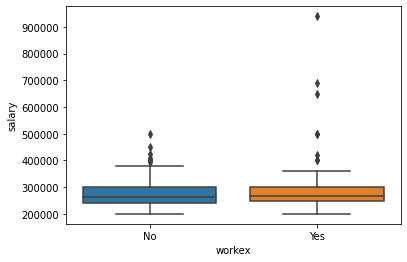figure , ax = plt.subplots(1,2 , figsize = (15,9))
df1 = df.loc[df['status'] == 'Placed']
sns.distplot(df1.loc[df['workex'] == 'Yes']['salary'] ,hist = False,ax = ax)
ax.set_title("Work Experience")
sns.distplot(df1.loc[df['workex'] == 'No']['salary']  , hist = False , ax = ax)
ax.set_title("No Work Experience")
ax.ticklabel_format(style = 'plain')
ax.ticklabel_format(style = 'plain')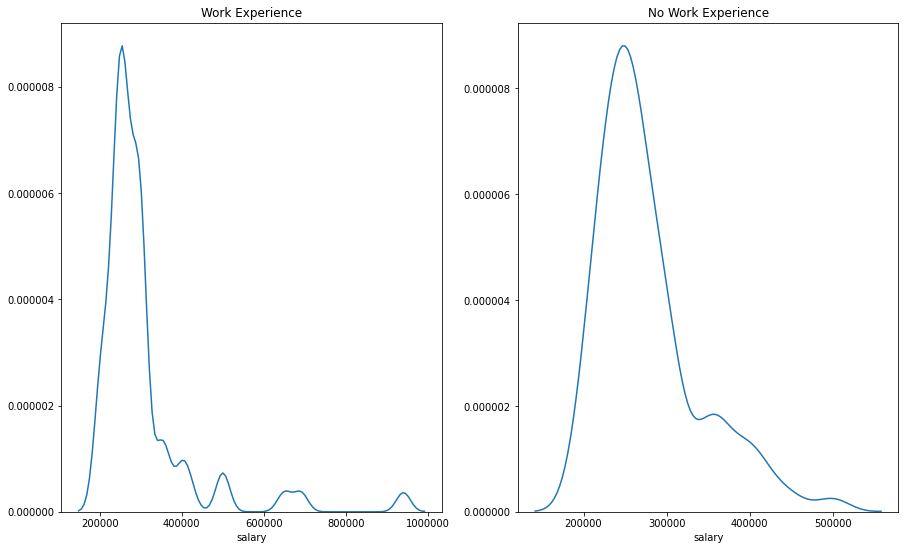Workex people have higher salaries as compared to no work experience.Hence we can say with work experience you can get a placement with more salary than with no work experience.

Lets see placement numbers for people from different ssc boards

df_boards_analysis =  df[['ssc_b','status']].groupby(['ssc_b'], as_index = False).count()
df_boards_analysis1 = df.loc[df['status'] == 'Placed'].groupby(['ssc_b'],as_index = False).count()
df_boards_analysis['Placed'] = df_boards_analysis1['status']
df_boards_analysis['Placed_Percent'] = df_boards_analysis['Placed']/df_boards_analysis['status']*100
df_boards_analysis.rename(columns = {'ssc_b':'SSC_Board' , 'status':'Total_Students'})

SSC_Board Total_Students Placed Placed_Percent
0 Central 116 78 67.241379
1 Others 99 70 70.707071

There is not much difference in placed percent so sscboard does not play an important role in deciding placement status.

sns.catplot(x = 'status' , y = 'ssc_p' , data = df )

<seaborn.axisgrid.FacetGrid at 0x7eff52546d68>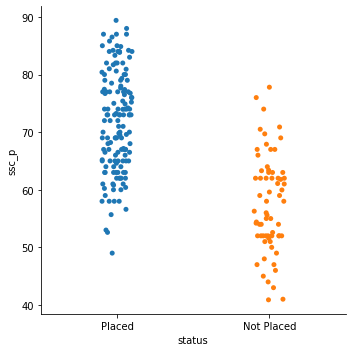Students who have more ssc percentage got placement .People below 50% were unplaced .

sns.boxplot(x = 'status' , y = 'ssc_p' , data = df )

<matplotlib.axes._subplots.AxesSubplot at 0x7eff5248f518>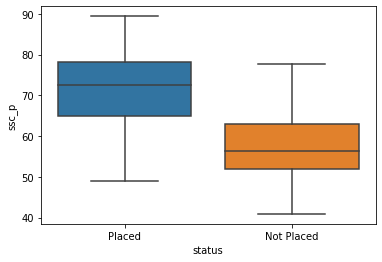We can see the same thing in box plot more clearly that ssc percentage influences placement

sns.boxplot(x = 'ssc_b' , y = 'salary' , data = df.loc[df['status']=='Placed'])

<matplotlib.axes._subplots.AxesSubplot at 0x7eff52402ef0>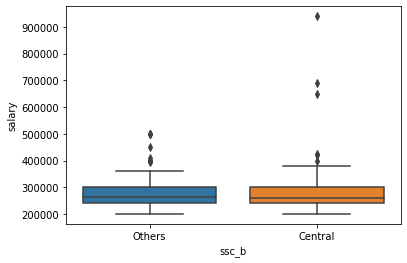Salaries are not that impacted from your ssc board . Although there are some outliers in central higher than others

df_hscboards_analysis =  df[['hsc_b','status']].groupby(['hsc_b'], as_index = False).count()
df_hscboards_analysis1 = df.loc[df['status'] == 'Placed'].groupby(['hsc_b'],as_index = False).count()
df_hscboards_analysis['Placed'] = df_hscboards_analysis1['status']
df_hscboards_analysis['Placed_Percent'] = df_hscboards_analysis['Placed']/df_hscboards_analysis['status']*100
df_hscboards_analysis.rename(columns = {'hsc_b':'HSC_Board' , 'status':'Total_Students'})

HSC_Board Total_Students Placed Placed_Percent
0 Central 84 57 67.857143
1 Others 131 91 69.465649

Same for hsc board it doesnt have much impact on placement as it is almost same for both boards.

sns.boxplot(x = 'hsc_b' , y = 'salary' , data = df.loc[df['status']== 'Placed'] )

<matplotlib.axes._subplots.AxesSubplot at 0x7eff523815c0>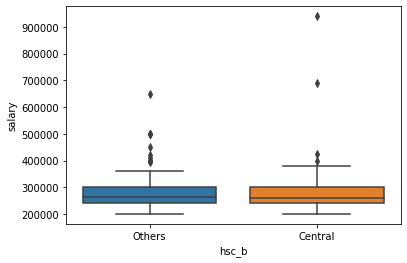sns.boxplot(x = 'status' , y = 'hsc_p' , data = df )

<matplotlib.axes._subplots.AxesSubplot at 0x7eff522fb6d8>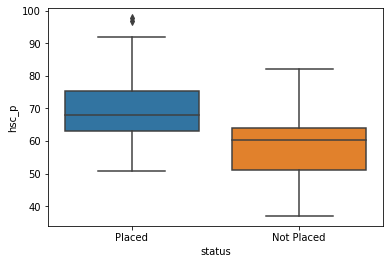HSc percentage is also impacting placement . More the value more likely the student is getting a placement.

df_hscsubject_analysis =  df[['hsc_s','status']].groupby(['hsc_s'], as_index = False).count()
df_hscsubject_analysis1 = df.loc[df['status'] == 'Placed'].groupby(['hsc_s'],as_index = False).count()
df_hscsubject_analysis['Placed'] = df_hscsubject_analysis1['status']
df_hscsubject_analysis['Placed_Percent'] = df_hscsubject_analysis['Placed']/df_hscsubject_analysis['status']*100
df_hscsubject_analysis.rename(columns = {'hsc_s':'HSC_subject' , 'status':'Total_Students'})

HSC_subject Total_Students Placed Placed_Percent
0 Arts 11 6 54.545455
1 Commerce 113 79 69.911504
2 Science 91 63 69.230769

Students from commerce and sccience background got more placements than arts.We can say HSC subject also has somewhat impact on placement

sns.boxplot(x = 'hsc_s' , y = 'salary' , data = df.loc[df['status']== 'Placed'] )

<matplotlib.axes._subplots.AxesSubplot at 0x7eff5227df98>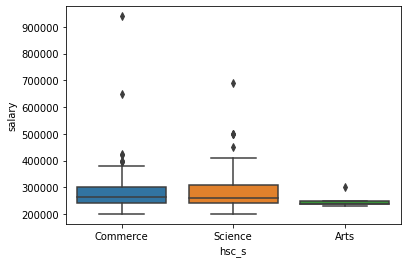sns.scatterplot(x = 'ssc_p', y = 'hsc_p' , data =df , hue = 'status')

<matplotlib.axes._subplots.AxesSubplot at 0x7eff52191518>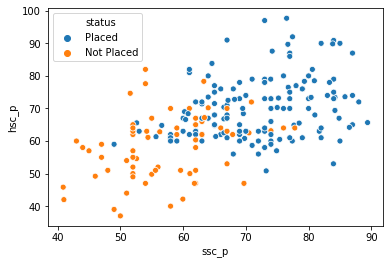sns.scatterplot(x = 'ssc_p', y = 'degree_p' , data =df , hue = 'status')

<matplotlib.axes._subplots.AxesSubplot at 0x7eff52127a58>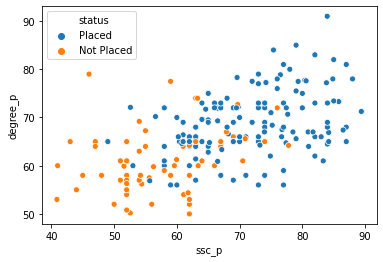sns.scatterplot(x = 'degree_p', y = 'hsc_p' , data =df , hue = 'status')

<matplotlib.axes._subplots.AxesSubplot at 0x7eff52098f28>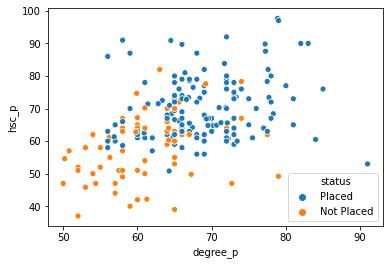people having more percentage tend to get placed than compared to unplaced.Be it in ssc , hsc or degree.Percentage matters for placement.

sns.countplot(x='degree_t' , data =df )

<matplotlib.axes._subplots.AxesSubplot at 0x7eff5216bd30>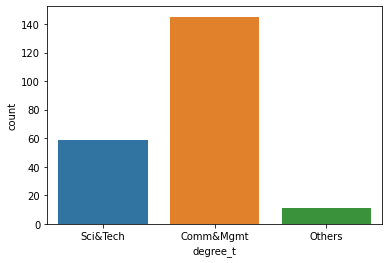If you see there is majority of people from commerce background .

df_degree_analysis =  df[['degree_t','status']].groupby(['degree_t'], as_index = False).count()
df_degree_analysis1 = df.loc[df['status'] == 'Placed'].groupby(['degree_t'],as_index = False).count()
df_degree_analysis['Placed'] = df_degree_analysis1['status']
df_degree_analysis['Placed_Percent'] = df_degree_analysis['Placed']/df_degree_analysis['status']*100
df_degree_analysis.rename(columns = {'degree_t':'Degree_Stream' , 'status':'Total_Students'})

Degree_Stream Total_Students Placed Placed_Percent
0 Comm&Mgmt 145 102 70.344828
1 Others 11 5 45.454545
2 Sci&Tech 59 41 69.491525

People from Science and Commerce have got the highest placements as compared to others.So Degree Stream does affect the placement

sns.boxplot(x = 'status' , y = 'degree_p' , data = df )

<matplotlib.axes._subplots.AxesSubplot at 0x7eff525f2198>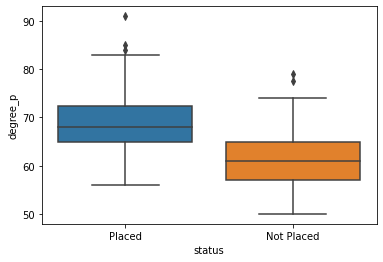More the degree percentage more is the probability of placement.

sns.scatterplot(x = 'degree_p' , y= 'salary' , data = df.loc[df['salary'] != 0])

<matplotlib.axes._subplots.AxesSubplot at 0x7eff52153f28>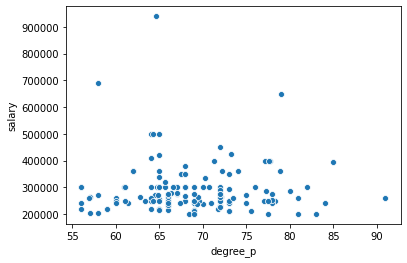We don't see any strong relation in degree_p and salary !!

sns.catplot(x = 'degree_t' , y = 'salary' , data = df.loc[df['salary'] != 0])

<seaborn.axisgrid.FacetGrid at 0x7eff5208b5c0>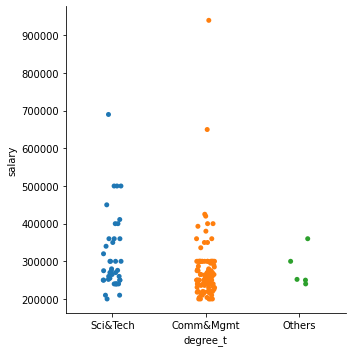sns.boxplot(x = 'degree_t' , y = 'salary' , data = df.loc[df['salary'] != 0])

<matplotlib.axes._subplots.AxesSubplot at 0x7eff51f060b8>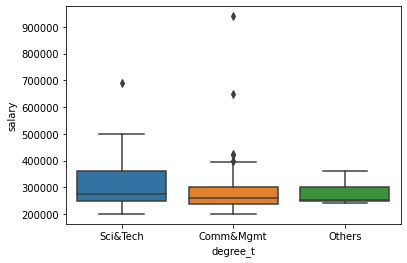Salaries for Sci&tech are higher than the other two streams.

sns.countplot(x = 'degree_t' , data =df , hue = 'gender')

<matplotlib.axes._subplots.AxesSubplot at 0x7eff51e1af28>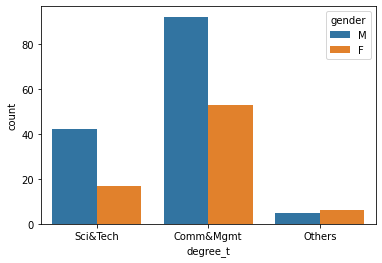Gender distribution is pretty good in others category as compared to sci and commerce
sns.catplot(x = 'degree_t' , y = 'salary' , data =df.loc[df['status']== 'Placed'] , hue = 'gender')

<seaborn.axisgrid.FacetGrid at 0x7eff51df7cf8>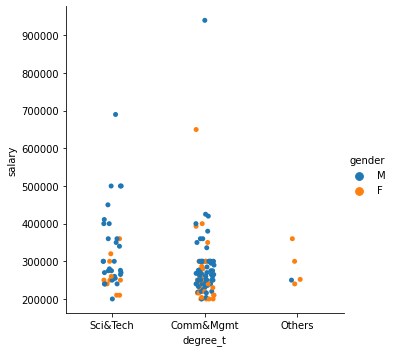sns.boxplot(x = 'degree_t' , y = 'salary', hue='gender' , data = df.loc[df['salary'] != 0])

<matplotlib.axes._subplots.AxesSubplot at 0x7eff51d7edd8>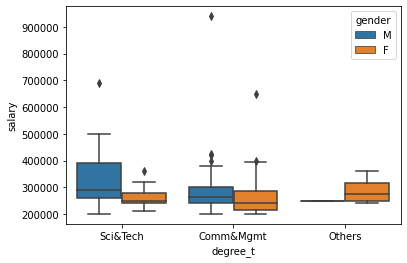For Science the salary range is bigger than other categories.Salaries are more in science as compared to commerce.

sns.regplot(x = 'mba_p', y = 'salary' , data = df)

<matplotlib.axes._subplots.AxesSubplot at 0x7eff51dfd940>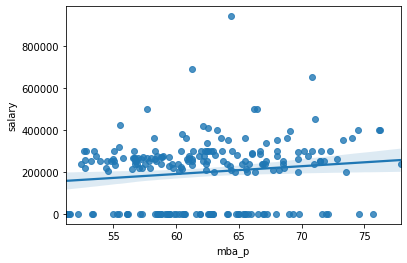There is no significant relation seen for mba percentage and salary.

sns.scatterplot(x = 'mba_p' , y = 'degree_p' , data = df , hue = 'status' )

<matplotlib.axes._subplots.AxesSubplot at 0x7eff51c3f898>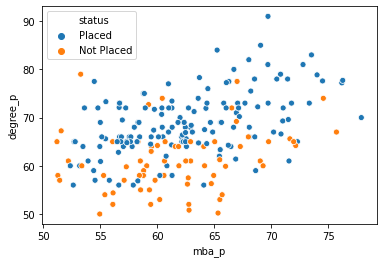sns.catplot(x = 'status' , y = 'etest_p' , data = df )

<seaborn.axisgrid.FacetGrid at 0x7eff51bf5438>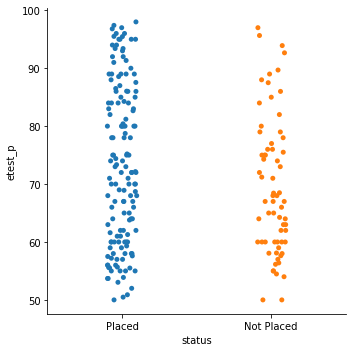Etest scores are not that different for placed and unplaced students.We can check mean score though.

df[['status','etest_p']].groupby('status').mean()

etest_p
status
Not Placed 69.587910
Placed 73.238041

As we can see mean is also almost same so Placement is not that affected by etest score

sns.regplot(x = 'etest_p' , y = 'salary' , data = df.loc[df['salary'] != 0])

<matplotlib.axes._subplots.AxesSubplot at 0x7eff51b8be48>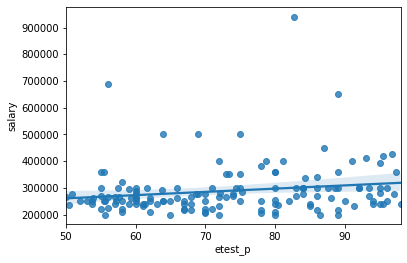The relation is not that significant for etest score and salary.

df_specialisation_analysis =  df[['specialisation','status']].groupby(['specialisation'], as_index = False).count()
df_specialisation_analysis1 = df.loc[df['status'] == 'Placed'].groupby(['specialisation'],as_index = False).count()
df_specialisation_analysis['Placed'] = df_specialisation_analysis1['status']
df_specialisation_analysis['Placed_Percent'] = df_specialisation_analysis['Placed']/df_specialisation_analysis['status']*100
df_specialisation_analysis.rename(columns = {'specialisation':'MBA_specialisation' , 'status':'Total_Students'})

MBA_specialisation Total_Students Placed Placed_Percent
0 Mkt&Fin 120 95 79.166667
1 Mkt&HR 95 53 55.789474

More students from Marketting and finance got placed as compared to Marketting and HR.There is more demand for the former specialisation.

sns.barplot(x = 'specialisation' , y = 'salary' , data =df.loc[df['status']== 'Placed'] , hue = 'gender')

<matplotlib.axes._subplots.AxesSubplot at 0x7eff51aeeef0>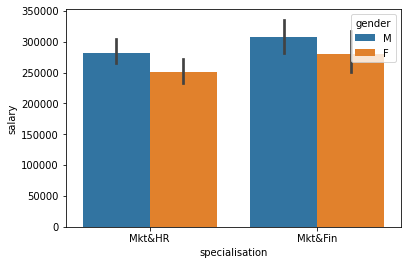Mean Salary difference is not that significant for different specialisation in MBA and even among genders.Thogh salaries are higher in finance as compared to HR and it also supports our observation above that demand is more in finance.Male employees earn more than females.

sns.catplot(x = 'specialisation' , y = 'salary' , data =df.loc[df['status']== 'Placed'] , hue = 'gender')

<seaborn.axisgrid.FacetGrid at 0x7eff51ae9978>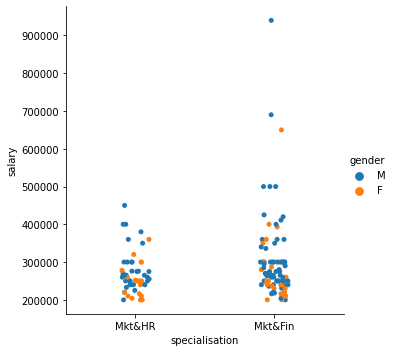Lets Do Label Encoding :

df = pd.get_dummies(df,columns = ['status'])
df = pd.get_dummies(df,columns = ['specialisation'])
df = pd.get_dummies(df,columns = ['gender'])
#df['status'] = le.fit_transform(df['status'])

#df_dummy.rename()

df.rename(columns = {'status_Not Placed':'Not Placed' , 'status_Placed':'Placed' ,
'specialisation_Mkt&Fin':'Marketting and Finance' , 'specialisation_Mkt&HR':'Marketting and HR',
'gender_F':'Female' , 'gender_M':'Male'}, inplace =True)

df.corrwith(df['Placed'])

sl_no                    -0.026859
ssc_p                     0.607889
hsc_p                     0.491228
degree_p                  0.479861
etest_p                   0.127639
mba_p                     0.076922
salary                    0.865774
Not Placed               -1.000000
Placed                    1.000000
Marketting and Finance    0.250655
Marketting and HR        -0.250655
Female                   -0.090670
Male                      0.090670
dtype: float64

SSC_percentage matters the most for getting a placement as it is highly correlated with Placement.

As we can see from above analysis , the factors affecting placement are : MBA specialisation , Degree Stream ,HSC_subject , Work Experience ,Degree percentage , ssc percentage ,Gender and hsc percentage

As per this dataset Males got more placement and higher salaries than females.

• MBA Specialization: Finance Department has higher placements and higher salaries
• Work Experience : People having workexperience are more likely of getting placed with higer salaries.
• Degree Percentage : Having more percentage can increase chances of placement but won't impact salary much
• Degree Stream : Science and commerce students get placed more as compared to arts .Salaries are higher for science than other streams.
• Having Higher HSC and SSC percentage can increase your chances of getting placed although boards don't play an important role in getting a placement
• Males are getting more placements and higher salaries than females.

Percentage matters for getting a placement.SSC percentage has greatest influence on placement